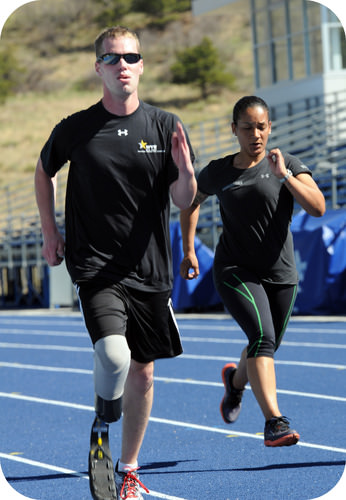# 2.5: Metric Unit Conversions

$$\newcommand{\vecs}{\overset { \rightharpoonup} {\mathbf{#1}} }$$ $$\newcommand{\vecd}{\overset{-\!-\!\rightharpoonup}{\vphantom{a}\smash {#1}}}$$$$\newcommand{\id}{\mathrm{id}}$$ $$\newcommand{\Span}{\mathrm{span}}$$ $$\newcommand{\kernel}{\mathrm{null}\,}$$ $$\newcommand{\range}{\mathrm{range}\,}$$ $$\newcommand{\RealPart}{\mathrm{Re}}$$ $$\newcommand{\ImaginaryPart}{\mathrm{Im}}$$ $$\newcommand{\Argument}{\mathrm{Arg}}$$ $$\newcommand{\norm}{\| #1 \|}$$ $$\newcommand{\inner}{\langle #1, #2 \rangle}$$ $$\newcommand{\Span}{\mathrm{span}}$$ $$\newcommand{\id}{\mathrm{id}}$$ $$\newcommand{\Span}{\mathrm{span}}$$ $$\newcommand{\kernel}{\mathrm{null}\,}$$ $$\newcommand{\range}{\mathrm{range}\,}$$ $$\newcommand{\RealPart}{\mathrm{Re}}$$ $$\newcommand{\ImaginaryPart}{\mathrm{Im}}$$ $$\newcommand{\Argument}{\mathrm{Arg}}$$ $$\newcommand{\norm}{\| #1 \|}$$ $$\newcommand{\inner}{\langle #1, #2 \rangle}$$ $$\newcommand{\Span}{\mathrm{span}}$$$$\newcommand{\AA}{\unicode[.8,0]{x212B}}$$Figure $$\PageIndex{1}$$ (Credit: Courtesy of the ​​​​​​​US Army; Source: http://commons.wikimedia.org/wiki/File:Flickr_-_The_U.S._Army_-_Track_practice.jpg(opens in new window); License: Public Domain)

### How can a number of track laps be converted to a distance in meters?

You are training for a 10-kilometer run by doing laps on a 400-meter track. You ask yourself how many times you need to run around the track in order to cover ten kilometers (...more than you realize). By using dimensional analysis, you can easily determine the number of laps needed to cover the $$10 \: \text{km}$$ distance.

## Metric Unit Conversions

The metric system's many prefixes allow quantities to be expressed in many different units. Dimensional analysis is useful to convert from one metric system unit to another.

##### Example $$\PageIndex{1}$$

A particular experiment for science class requires $$120 \: \text{mL}$$ of a solution. The science teacher knows that he will need to make enough solution for 40 experiments to be performed throughout the day. How many liters of solution should he prepare?

###### Known
• 1 experiment requires $$120 \: \text{mL}$$
• $$1 \: \text{L} = 1000 \: \text{mL}$$
###### Unknown

Since each experiment requires $$120 \: \text{mL}$$ of solution and the teacher needs to prepare enough for 40 experiments, multiply 120 by 40 to get $$4800 \: \text{mL}$$ of solution needed. Now you must convert $$\text{mL}$$ to $$\text{L}$$ by using a conversion factor.

###### Step 2: Calculate.

$4800 \: \text{mL} \times \frac{1 \: \text{L}}{1000 \: \text{mL}} = 4.8 \: \text{L}\nonumber$

Note that the conversion factor is arranged so that the $$\text{mL}$$ unit is in the denominator and thus cancels out, leaving $$\text{L}$$ as the remaining unit in the answer.

A liter is much larger than a milliliter, so it makes sense that the number of liters required is less than the number of milliliters.

## Two-Step Metric Unit Conversions

Some metric conversion problems are most easily solved by breaking them down into more than one step. When both the given unit and the desired unit have prefixes, one can first convert to the simple (un-prefixed) unit, followed by a conversion to the desired unit. The following example will illustrate this method.

##### Example $$\PageIndex{2}$$

Convert $$4.3 \: \text{cm}$$ to $$\mu \text{m}$$.

###### Known
• $$1 \: \text{m} = 100 \: \text{cm}$$
• $$1 \: \text{m} = 10^6 \: \mu \text{m}$$
###### Unknown

You may need to consult a table for the multiplication factor represented by each metric prefix. First convert $$\text{cm}$$ to $$\text{m}$$, then convert $$\text{m}$$ to $$\mu \text{m}$$.

###### Step 2: Calculate.

$4.3 \: \text{cm} \times \frac{1 \: \text{m}}{100 \: \text{cm}} \times \frac{10^6 \: \mu \text{m}}{1 \: \text{m}} = 43,000 \: \mu \text{m}\nonumber$

Each conversion factor is written so that the unit of the denominator cancels with the unit of the numerator of the previous factor.

A micrometer is a smaller unit of length than a centimeter, so the answer in micrometers is larger than the number of centimeters given.

## Summary

• Dimensional analysis can be used to carry out metric unit conversions.

## Review

1. Perform the following conversions.
1. 0.074 km to m
2. 24,600 μg to g
3. 4.9 × 107 μg to kg
4. 84 dm to mm

2.5: Metric Unit Conversions is shared under a CC BY-NC license and was authored, remixed, and/or curated by LibreTexts.